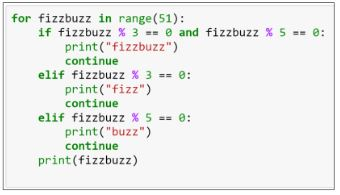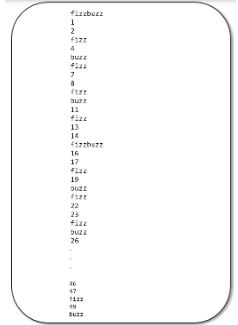In your choice of language, write a program that prints the numbers ranging from one to 50.

Q:
In your choice of language, write a program that prints the numbers ranging from one to 50.

But for multiples of three, print "Fizz" instead of the number, and for the multiples of five, print "Buzz." For numbers which are multiples of both three and five, print "FizzBuzz"

The code is shown below:Note that the range mentioned is 51, which means zero to 50. However, the range asked in the question is one to 50. Therefore, in the above code, you can include the range as (1,51).

The output of the above code is as shown:+1 vote